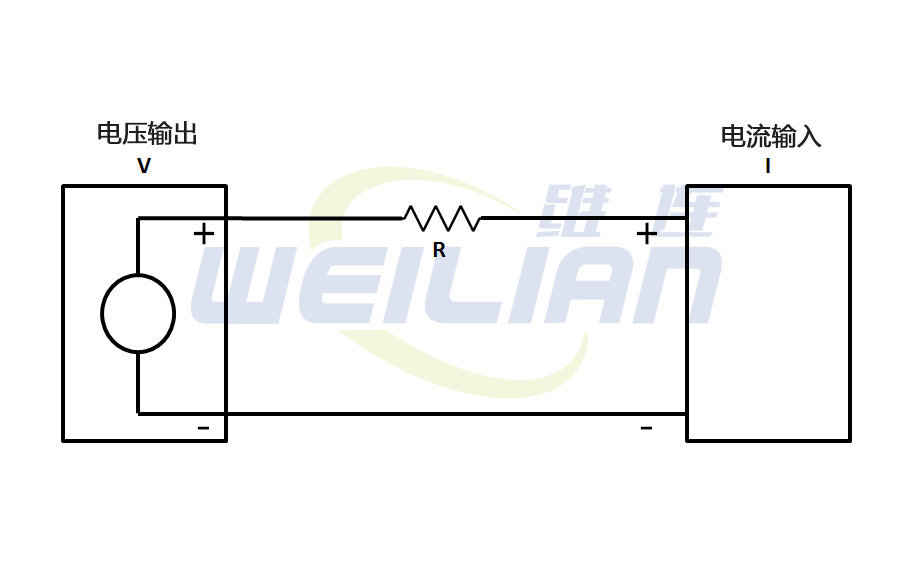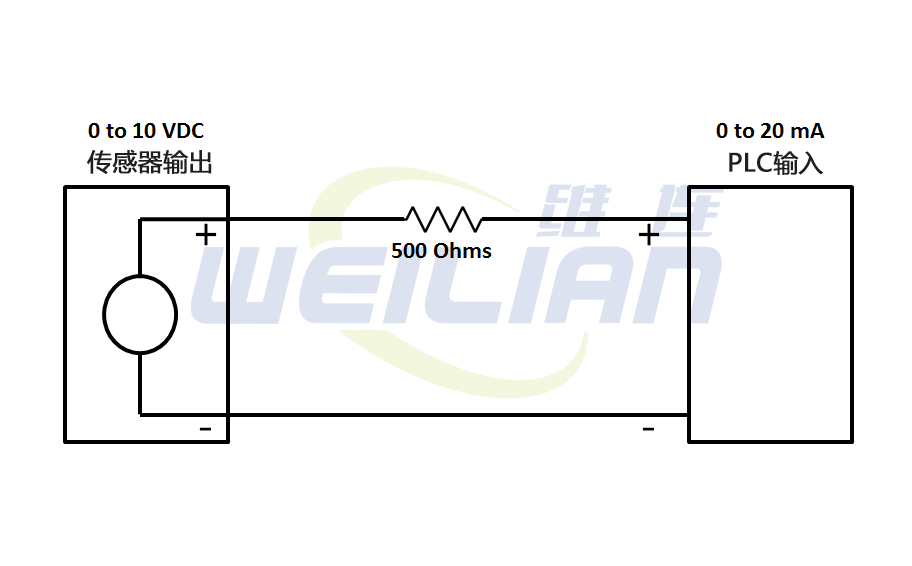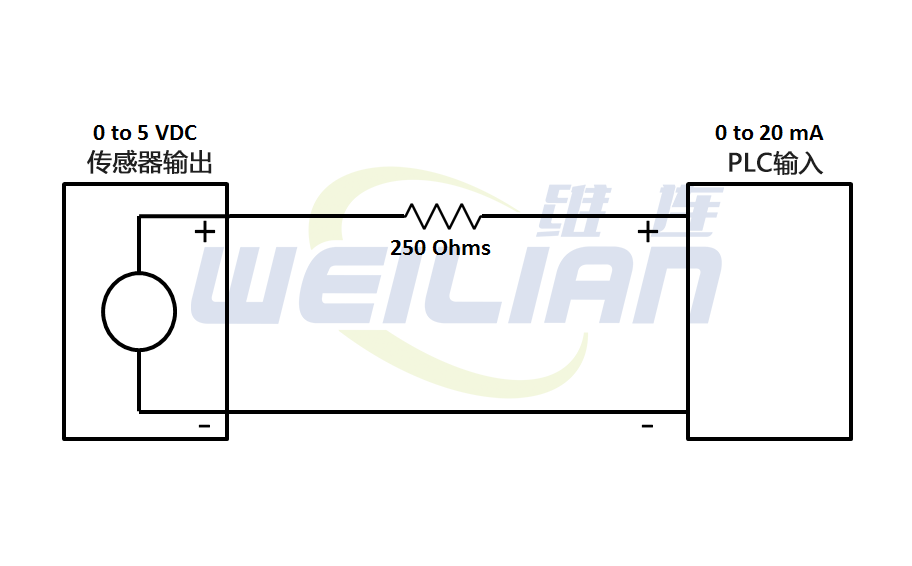### 如何使用电阻将电压转换为电流？

2021-09-14       来源：www.weilianchina.comR = 10V/0.020A = 500 欧姆I = V/R = 0/500 = 0mA

I = V/R = 10/500 = 0.02A = 20mA

R = 5V/0.020A = 250 欧姆I = V/R = 0/250 = 0mA
I= V/R = 5/250 = 0.02A = 20mA维连在线客服
>
Top